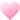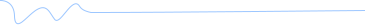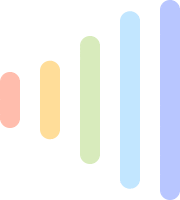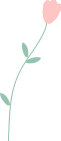520

• 微信客服

xmyeditor2021• QQ群

837641332• 排版互赞交流群

回复【排版】进群• 各行业运营群

回复【运营】进群•• 标题

• 基础标题

• 线框标题

• 编号标题

• 底色标题

• 图片标题

• 主副标题

• 竖列标题

• 全部标题

• 10W+素材

• 内容

• 基础布局

• 基础内容

• 标题内容

• 线条边框

• 图片边框

• 小图内容

• 纯色内容

• 条纹背景

• 动态背景

• 信纸样式

• 其它背景

• 全部内容

• 10W+素材

• 图片

• 基础图片

• 单图样式

• 圆形样式

• 双图组合

• 上下组合

• 左右组合

• 三图组合

• 四图组合

• 多图组合

• 封面大图

• 全部图片

• 10W+素材

• 分割

• 简约线条

• 卡通动物

• 花草树木

• 颜色可变

• 动态小图

• 人物其它

• End结语

• 全部分割

• 10W+素材

• 二维码

• 静态背景

• 动态背景

• 纯色类型

• 线框卡片

• 指纹

• 其它码

• 全部码

• 10W+素材

• 引导

• 顶部关注

• 开篇引言

• 往期回顾

• 在看引导

• 分享引导

• 头条关注

• 阅读原文

• 点赞引导

• 全部引导

• 10W+素材

• 节日

• 儿童节

• 端午节

• 父亲节

• 建党节

• 建军节

• 七夕节

• 教师节

• 中秋节

• 国庆节

• 重阳节

• 万圣节

• 光棍节

• 感恩节

• 圣诞节

• 腊八节

• 元旦

• 春节

• 秋天

• 冬季

• 春运

• 元宵节

• 夏日

• 春天

• 电商节

• 母亲节

• 护士节

• 24节气

• 青年节

• 51劳动节

• 清明节

• 愚人节

• 315

• 植树节

• 女神节

• 全部节日

• 10W+素材

• 主题

• 古风怀旧

• 简约文字

• 文艺清新

• 人物介绍

• 访谈演讲

• 活动宣传

• 祈福公益

• 聚会庆祝

• 全部标题

• 10W+素材

• 组件

• 视频样式

• 音频样式

• 电话地址

• 数据表格

• 对话问答

• 轴线排序

• 滑动样式

• 表情符号

• 微信标题

• 表情相关

• 人物相关

• 植物自然

• 动物系列

• 生肖星座

• 水果食物

• 花样庆祝

• 运动休闲

• 房车交通

• 文字系列

• 各种标示

• 物体物件

• 国旗旗帜

• svg交互

• 加点料

• 猜成语

• 爆笑

• 整人

• 经典

• 高智商

• 推理

• 侦探

• 恐怖

• 图片

• 猜名字

• 打一物

• 字谜

• 全部料

• 箭头

• 按钮

• 搞怪

• 全部组件

• 10W+素材

• 行业

• 医疗健康

• 公检司法

• 行政政务

• 旅游出行

• 餐饮美食

• 物业社区

• 中学·大学

• 幼儿·小学

• 电商购物

• 汽车交通

• 科技商务

• 警察警务

• 成人教育

• 文娱影视

• 金融理财

• 美妆穿搭

• 佛道修禅

• 爱情婚礼

• 体育运动

• 萌宠宠物

• 酒店房产

• 安保消防

• 农林牧渔

• 游戏竞技

• 档案图书

• 气象天气

• 飞机航空

• 铁路高铁

• 全部行业

• 10W+素材

• 收藏时间
• 使用时间
• 使用次数

• 免费
• VIP
• 全部Dear:ENDYOU ARE ROMAC关 闭

• 复制

复制

• 撤销

撤销

• 保存

保存

• 清空

清空

• 草稿

草稿

• 预览

预览

• 同步

同步

• 导出

导出

• 采集

采集• 我的图片

• 无版权图

无版权图

GIF动图

表情图

全选
删除
批量删除

移动分组

折叠美图
拼图
修图

15秒后自动关闭本窗口

我知道了，今日不再提示了！

推荐小图我的图标

默认分组

•确定删除此内容吗？

取消 确认

样式展示区

在这里选择排版样式

1/6

下一步